# Edges

#### 2022-08-08

If the natural `ggplot2` equivalent to nodes is `geom_point()`, then surely the equivalent to edges must be `geom_segment()`? Well, sort of, but there’s a bit more to it than that.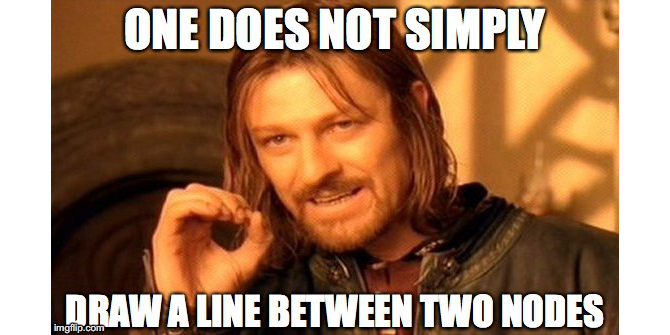One does not simply draw a line between two nodes

While nodes are the sensible, mature, and predictably geoms, edges are the edgy (sorry), younger cousins that pushes the boundaries. To put it bluntly:

On the ggraph savannah you definitely want to be an edge!

## Meet the `geom_edge_*()` family

While the introduction might feel a bit over-the-top it is entirely true. An edge is an abstract concept denoting a relationship between two entities. A straight line is simply just one of many ways this relationship can be visualised. As we saw when discussing nodes sometimes it is not drawn at all but impied using containment or position (treemap, circle packing, and partition layouts), but more often it is shown using a line of some sort. This use-case is handled by the large family of edge geoms provided in `ggraph`. Some of the edges are general while others are dedicated to specific layouts. Let’s creates some graphs for illustrative purposes first:

``````library(ggraph)
library(tidygraph)
library(purrr)
library(rlang)

set_graph_style(plot_margin = margin(1,1,1,1))
hierarchy <- as_tbl_graph(hclust(dist(iris[, 1:4]))) %>%
mutate(Class = map_bfs_back_chr(node_is_root(), .f = function(node, path, ...) {
if (leaf[node]) {
as.character(iris\$Species[as.integer(label[node])])
} else {
species <- unique(unlist(path\$result))
if (length(species) == 1) {
species
} else {
NA_character_
}
}
}))``````
``````## Warning in bfs(graph = graph, root = root, neimode = mode, unreachable =
## unreachable, : Argument `neimode' is deprecated; use `mode' instead``````
``````hairball <- as_tbl_graph(highschool) %>%
mutate(
year_pop = map_local(mode = 'in', .f = function(neighborhood, ...) {
neighborhood %E>% pull(year) %>% table() %>% sort(decreasing = TRUE)
}),
pop_devel = map_chr(year_pop, function(pop) {
if (length(pop) == 0 || length(unique(pop)) == 1) return('unchanged')
switch(names(pop)[which.max(pop)],
'1957' = 'decreased',
'1958' = 'increased')
}),
popularity = map_dbl(year_pop, ~ .) %|% 0
) %>%
activate(edges) %>%
mutate(year = as.character(year))``````

### Fan

Sometimes the graph is not simple, i.e. it has multiple edges between the same nodes. Using links is a bad choice here because edges will overlap and the viewer will be unable to discover parallel edges. `geom_edge_fan()` got you covered here. If there are no parallel edges it behaves like `geom_edge_link()` and draws a straight line, but if parallel edges exists it will spread them out as arcs with different curvature. Parallel edges will be sorted by directionality prior to plotting so edges flowing in the same direction will be plotted together:

``````ggraph(hairball, layout = 'stress') +
geom_edge_fan(aes(colour = year))``````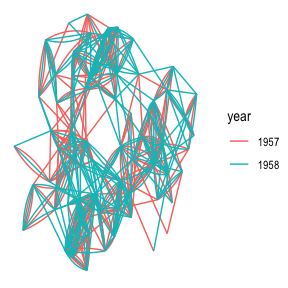### Parallel

An alternative to `geom_edge_fan()` is `geom_edge_parallel()`. It will draw edges as straight lines but in the case of multi-edges it will offset each edge a bit so they run parallel to each other. As with `geom_edge_fan()` the edges will be sorted by direction first. The offset is done at draw time and will thus remain constant even during resizing:

``````ggraph(hairball, layout = 'stress') +
geom_edge_parallel(aes(colour = year))``````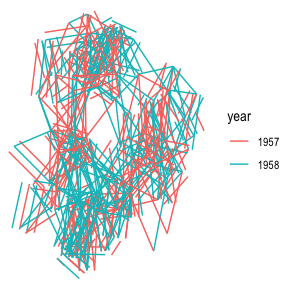### Loops

Loops cannot be shown with regular edges as they have no length. A dedicated `geom_edge_loop()` exists for these cases:

``````# let's make some of the student love themselves
loopy_hairball <- hairball %>%
bind_edges(tibble::tibble(from = 1:5, to = 1:5, year = rep('1957', 5)))
ggraph(loopy_hairball, layout = 'stress') +
geom_edge_link(aes(colour = year), alpha = 0.25) +
geom_edge_loop(aes(colour = year))``````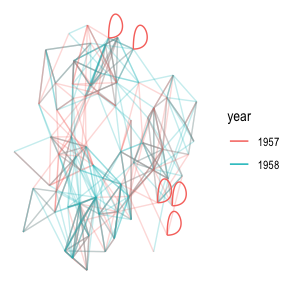The direction, span, and strength of the loop can all be controlled, but in general loops will add a lot of visual clutter to your plot unless the graph is very simple.

### Density

This one is definitely strange, and I’m unsure of it’s usefulness, but it is here and it deserves an introduction. Consider the case where it is of interest to see which types of edges dominates certain areas of the graph. You can colour the edges, but edges can tend to get overplotted, thus reducing readability. `geom_edge_density()` lets you add a shading to your plot based on the density of edges in a certain area:

``````ggraph(hairball, layout = 'stress') +
geom_edge_density(aes(fill = year)) +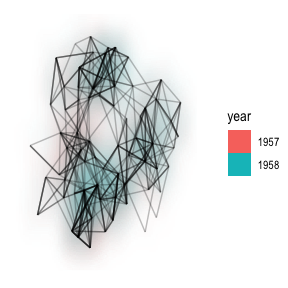### Arcs

While some insists that curved edges should be used in standard “hairball” graph visualisations it really is a poor choice, as it increases overplotting and decreases interpretability for virtually no gain (unless complexity is your thing). That doesn’t mean arcs have no use in graph visualizations. Linear and circular layouts can benefit greatly from them and `geom_edge_arc()` is provided precisely for this scenario:

``````ggraph(hairball, layout = 'linear') +
geom_edge_arc(aes(colour = year))``````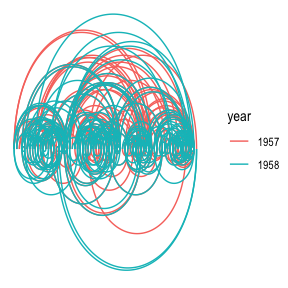Arcs behave differently in circular layouts as they will always bend towards the center no matter the direction of the edge (the same thing can be achieved in a linear layout by setting `fold = TRUE`).

``````ggraph(hairball, layout = 'linear', circular = TRUE) +
geom_edge_arc(aes(colour = year)) +
coord_fixed()``````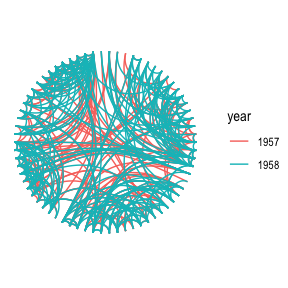### Elbow

Aah… The classic dendrogram with its right angle bends. Of course such visualizations are also supported with the `geom_edge_elbow()`. It goes without saying that this type of edge requires a layout that flows in a defined direction, such as a tree:

``````ggraph(hierarchy, layout = 'dendrogram', height = height) +
geom_edge_elbow()``````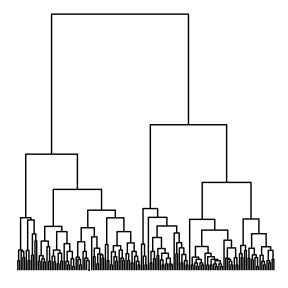### Diagonals

If right angles aren’t really your thing `ggraph` provides a smoother version in the form of `geom_edge_diagonal()`. This edge is a quadratic bezier with control points positioned at the same x-value as the terminal nodes and halfway in-between the nodes on the y-axis. The result is more organic than the elbows:

``````ggraph(hierarchy, layout = 'dendrogram', height = height) +
geom_edge_diagonal()``````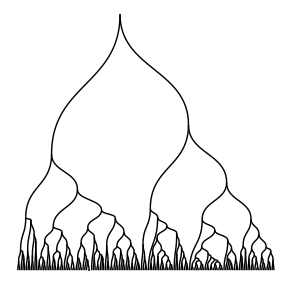It tends to look a bit weird with hugely unbalanced trees so use with care…

### Bends

An alternative to diagonals are bend edges which are elbow edges with a smoothed corner. It is implemented as a quadratic bezier with control points at the location of the expected elbow corner:

``````ggraph(hierarchy, layout = 'dendrogram', height = height) +
geom_edge_bend()``````